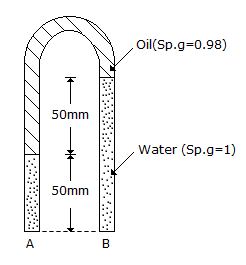# Civil Engineering - UPSC Civil Service Exam Questions - Discussion

48.

In the set up shown in the above figure, assuming the specific weight of water as 10, 000 N/m3, the pressure difference between the points A and B will be[A]. 10 N/m2 [B]. - 10 N/m2 [C]. 20 N/m2 [D]. -20 N/m2

Explanation:

No answer description available for this question.

 Hema N said: (Aug 28, 2017) Pressure due to water=10000*1*9.81*0.05 = 490.5. Pressure due to oil=10000*0.98*9.81*0.05 = 480.5. Diff=480.5-490.5 = -10.

 Jinish said: (Jan 20, 2020) Pressure = density * gravity * height.

 Amit Singh said: (Jan 31, 2021) Pa-1 * 10000 * 50 * 10^(-3)-0.98 * 10000 * 50 * 10^(-3) = Pb-1 * 10000 * 100 * 10^(-3). Pa - Pb = -10.

 Lalit Kumar said: (Mar 11, 2021) Pressure due to water=10000*1*0.05 = 500, Pressure due to oil=10000*0.98*0.05 = 490, Diff=490-500 = -10. It is already a specific weight no need to multiply by 9.81.

 Divesh Kumar said: (Sep 5, 2021) Pa-10000 *.050-.98 * 10000 * .050 = Pb-10000 * .100. Pa-Pb = 500+490-1000. Pa-Pb = -10N/m^2.